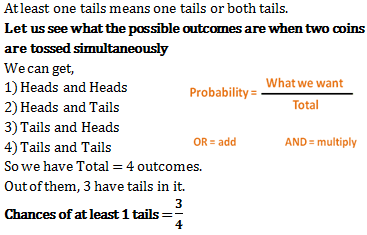Courses

# Probability - Olympiad Level MCQ, Class 9 Mathematics

## 22 Questions MCQ Test Olympiad Preparation for Class 9 | Probability - Olympiad Level MCQ, Class 9 Mathematics

Description
This mock test of Probability - Olympiad Level MCQ, Class 9 Mathematics for Class 9 helps you for every Class 9 entrance exam. This contains 22 Multiple Choice Questions for Class 9 Probability - Olympiad Level MCQ, Class 9 Mathematics (mcq) to study with solutions a complete question bank. The solved questions answers in this Probability - Olympiad Level MCQ, Class 9 Mathematics quiz give you a good mix of easy questions and tough questions. Class 9 students definitely take this Probability - Olympiad Level MCQ, Class 9 Mathematics exercise for a better result in the exam. You can find other Probability - Olympiad Level MCQ, Class 9 Mathematics extra questions, long questions & short questions for Class 9 on EduRev as well by searching above.
QUESTION: 1

### When two coins are tossed simultaneously, what are the chances of getting at least one tail?

Solution:QUESTION: 2

Solution:
QUESTION: 3

### The probability of getting a multiple of 3 is

Solution: The question is incomplete.It will be like this An unbiased die is tossed.Find the probability of getting a multiple of 3.Sol:Here S = {1,2,3,4,5,6}
Let E be the event of getting the multiple of 3
Then, E = {3,6}
P(E) = n(E)/n(S) = 2/6 = 1/3
Hope u got it.....
QUESTION: 4

The probability of getting a number greater than 1 is

Solution:
QUESTION: 5

The probability of getting a number between 1 and 6 is

Solution:
QUESTION: 6

The probability of getting an odd number is

Solution:
QUESTION: 7

The probability of getting one head is

Solution:
QUESTION: 8

The probability of getting two heads

QUESTION: 9

The probability of getting no head is

Solution:
QUESTION: 10

The probability of getting at least one head is

Solution:
When  tossing two coins simultaneously then the possible of outcomes are: (HH) or (HT) or (TH)  or (TT) respectively; where H is denoted for head and T is denoted for tail.

Therefore, total numbers of outcome are 22 = 4.

Probability of getting at least 1 head.

Let E be event of getting at least 1 head.

Then, E = {(HH , HT, TH}

Therefore, n(E) = 3.

Therefore, P(getting at least 1 head) = P(E) = n(E)/n(S) = 3/4.
QUESTION: 11

The probability of getting a jack card is

Solution:
QUESTION: 12

The probability of getting a face card is

Solution: There are 12 face cards in the deck of 52 cards...so the probability of getting a face card is 12÷52 = 3/13
QUESTION: 13

The probability of getting a '10' of black suit is

Solution:
QUESTION: 14

The probability of getting a red and a king card is

Solution: Total Red Cards = 26
Total King cards = 4
Total red and King cards = 28 because two king cards are already included in red cards
therefore probabilty = 28/52
= 7/13
QUESTION: 15

A bag contains 4 red balls and 3 green balls. A ball is drawn at random. The probability of drawing a green ball is

Solution:
QUESTION: 16

P(E) + P(E)¯ is equal to

Solution:
QUESTION: 17

Which one of the following cannot be the probability of an event

Solution:
QUESTION: 18

Which one of the following cannot be the probability of an event

Solution:
QUESTION: 19

Probability of an impossible event is equal to

Solution:
QUESTION: 20

If P(E1) =1/6, P(E2) =1/3, P(E3) =1/6, where E1, E2, E3 and E4 are elementary events of a random experiment,then P(E4) is equal to

Solution:
QUESTION: 21

Cards each marked with one of the numbers 4, 5, 6,...., 20 are placed in a box and mixed thoroughly. One card is drawn at random from the box. Then, the probability of getting an even prime number is

Solution:
QUESTION: 22

A bag contains 5 red and 4 black balls. A ball is drawn at random from the bag. Then, the probability of getting a black ball is

Solution: